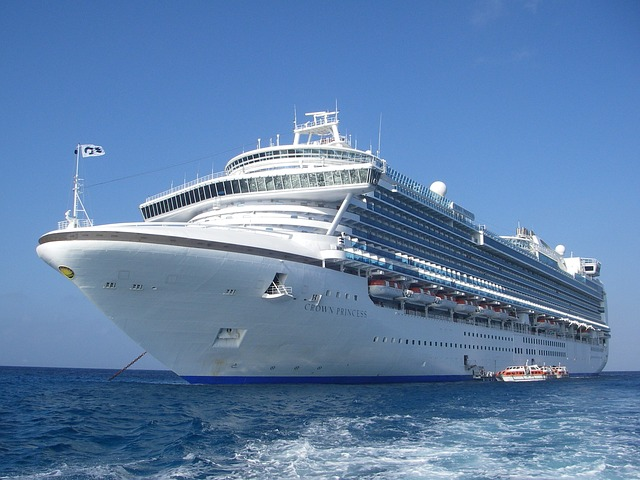Edexcel GCSE - Waves 2

page 1

Waves topics in the physics (triple science) syllabus - not for combined/double science students.

1. What is the highest frequency humans can hear?

• A). 10 kHz
• B). 20 kHz
• C). 100 kHz
• D). 200 kHz

2. What is the lowest frequency humans can hear?

• A). 10 Hz
• B). 20 Hz
• C). 100 Hz
• D). 200 Hz

3. Which of the answers below gives the correct name for sound waves with a higher and lower frequency than human hearing?

 above human hearing below human hearing A megasound microsound B megasound infrasound C ultrasound microsound D ultrasound infrasound

4. Dolphins and submarines use very high frequency sound waves to find the location of objects under the oceans. This technique is called...

• B). pulse scanning
• C). microwave location
• D). sonar

5. When sound waves move from water into air, they slow down. Which statement below explains the changes, if any, to the frequency and wavelength of sound waves in this situation?

• A). Wavelength increases, frequency decreases.
• B). Wavelength decreases, frequency decreases.
• C). Wavelength stays the same, frequency decreases.
• D). Wavelength decreases, frequency stays the same.

6. A boat sends a high freqency pulse to the bottom of the ocean. It travels at 1500 m/s through the sea water. If the pulse echo is detected 4 seconds later, how deep is the ocean at this point?

• A). 3 km
• B). 4 km
• C). 5 km
• D). 6 km7. Which of these reflected light rays has been drawn correctly?8.Reflection in a single direction, as shown in question 7, is called....

• A). diffuse reflection.
• B). external reflection.
• C). specular reflection.
• D). linear reflection.